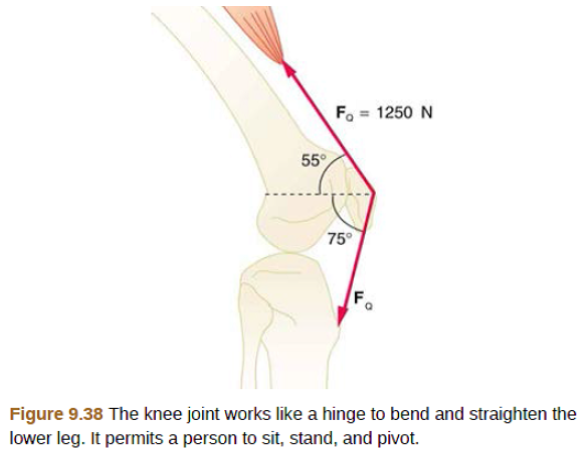# Problem: The upper leg muscle (quadriceps) exerts a force of 1250 N, which is carried by a tendon over the kneecap (the patella) at the angles shown in Figure 9.38. Find the direction and magnitude of the force exerted by the kneecap on the upper leg bone (the femur).

###### FREE Expert Solution

Vector Magnitude:

$\overline{)\mathbf{|}\stackrel{\mathbf{⇀}}{\mathbit{A}}\mathbf{|}{\mathbf{=}}\sqrt{{{\mathbit{A}}_{\mathbit{x}}}^{\mathbf{2}}\mathbf{+}{{\mathbit{A}}_{\mathbit{y}}}^{\mathbf{2}}}}$

Vector Direction:

$\overline{){\mathbf{tan}}{\mathbit{\theta }}{\mathbf{=}}\frac{{\mathbit{A}}_{\mathbit{y}}}{{\mathbit{A}}_{\mathbit{x}}}}$

Vector Components:

The component of the force in x-direction is:

Fx = (1250)cos(55°) + (1250)cos(75°) = 1040N

86% (122 ratings)###### Problem Details

The upper leg muscle (quadriceps) exerts a force of 1250 N, which is carried by a tendon over the kneecap (the patella) at the angles shown in Figure 9.38. Find the direction and magnitude of the force exerted by the kneecap on the upper leg bone (the femur).